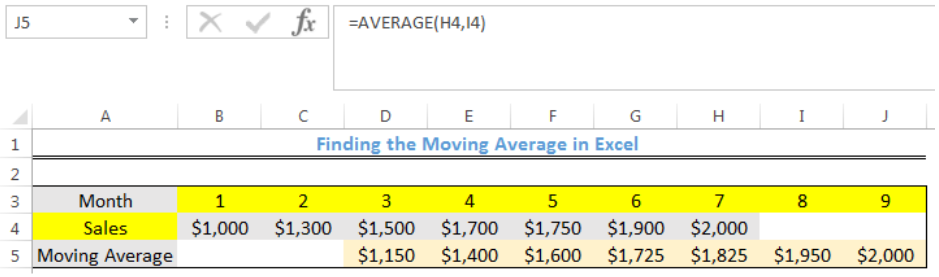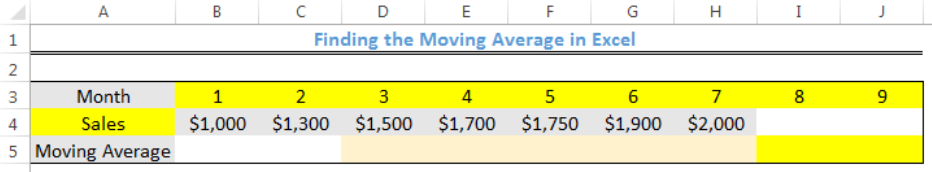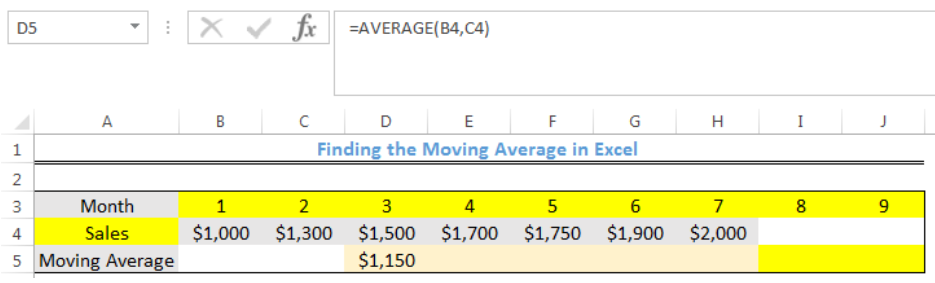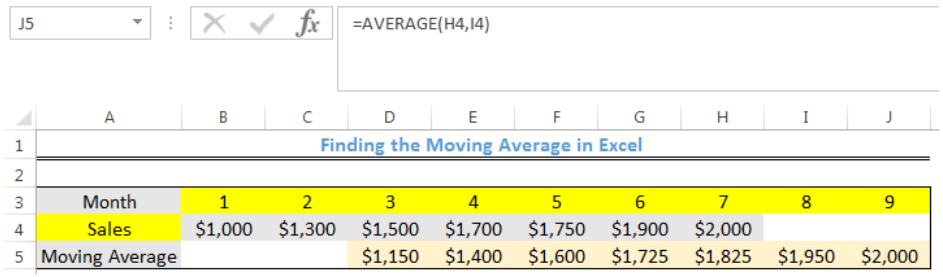Get instant live expert help with Excel or Google Sheets“My Excelchat expert helped me in less than 20 minutes, saving me what would have been 5 hours of work!”

#### Post your problem and you’ll get expert help in seconds.

Your message must be at least 40 characters
Our professional experts are available now. Your privacy is guaranteed.

# Here’s a Guide on Finding the Moving Average in Excel

We can use the AVERAGE function to FIND THE MOVING AVERAGE OF A DATA. Calculation of moving averages is useful for financial forecasting. The steps below will walk through the process.Figure 1- Using Moving Average for Forecasting Sales for Month 8 and 9

## Syntax

`=AVERAGE(RANGE)`

• RANGE refers to the cell references that corresponds to the numbers in each cell

## Formula

`=AVERAGE(B4,C4)`

## Setting up the Data

With the data in figure 2, we will make a forecast of sales for the next two months. We will use the moving average for every 2 months to calculate the forecast for month 8 and 9.

• We will enter the Months in Row 3
• We will enter the Sales in Row 4
• Our result for moving average for every 2 months will be returned in Row 5Figure 2 – Setting up the Data

## Calculate the MOVING AVERAGE FOR EVERY 2 MONTHS

• We will click on Cell D5
• We will insert the formula below into Cell D5
`=AVERAGE(B4,C4)`
• We will press the enter keyFigure 3- Moving Average for the First two months

The average value taken is that of Cell B4 and Cell C4. The next average value will be of Cell C4 and Cell D4 and so on.

• We will click on Cell D5 again
• We will double click on the fill handle tool which is the small plus sign you see at the bottom right of Cell D5. Select and drag the formula across the row to Cell J5 to copy the formula to the other cells.Figure 4- Forecast for Month 8 and 9 by Moving Average

If we were to calculate the moving average for every 3 months with the same data, we will use the formula below:

`=AVERAGE(B4:D4)`

We will click on Cell E5 and insert the formula rather than D5 when working with 2 months sales or 2-month moving average.

## Instant Connection to an Expert through our Excelchat Service

Most of the time, the problem you will need to solve will be more complex than a simple application of a formula or function. If you want to save hours of research and frustration, try our live Excelchat service! Our Excel Experts are available 24/7 to answer any Excel question you may have. We guarantee a connection within 30 seconds and a customized solution within 20 minutes.

Are you still looking for help with the Average function? View our comprehensive round-up of Average function tutorials here.

Solution examplesOn the Grade Average worksheet, select G7 and type "Average." In cell G8, use a formula to generate the average of the contents in Cells B8:F8. Use the fill handle to populate your results through G10. The syntax for the Excel Average function is the following: AVERAGE(argument1, [argument2],...). Arguments can be numbers, named ranges, cell references that contain numbers, or arrays. Select Cell A12, and enter "Class Average." Use a formula to generate the average of IP Assignment 1 in cell B12. Use the fill across handle to populate your results of averaging the other assignments to F12
Solved by Z. A. in 40 minsI need help calculating average time elapsed. It is in dd:hh:mm:ss text format and so I can't calculate using =AVERAGEIF
Solved by O. E. in 11 minsI contacted you yesterdaya bout how to use the =AVERAGE function, and for real numbers it works fine, but I tried to get the expert to explain to me how to use the =AVERAGE function when the cells have formulas in them that generate '0' value, so the AVERAGE function would ignore these cells in the averaging method, but got no answer. So, I need to know how use the =AVERAGE function so that when there are formulas in the selected cells to average, that EXCEL would ignore those cells like they have real numbers in them, which seems to work fine. Please advise...
Solved by C. J. in 30 minsI need an IF formula where, IF there is a manual adjustment in a whole column of 100% values (say someone changes one to 90%) would say "Yes" or "No". Tried to solve this with =IF(AVERAGE(...)=100%, "No", "Yes") but it is kind of sloppy).
Solved by M. H. in 20 minsIF(AVERAGE(C6+E6+G6)>=5000,TRUE,FALSE) I am using AND function and it is not giving me the correct answer
Solved by G. J. in 24 mins